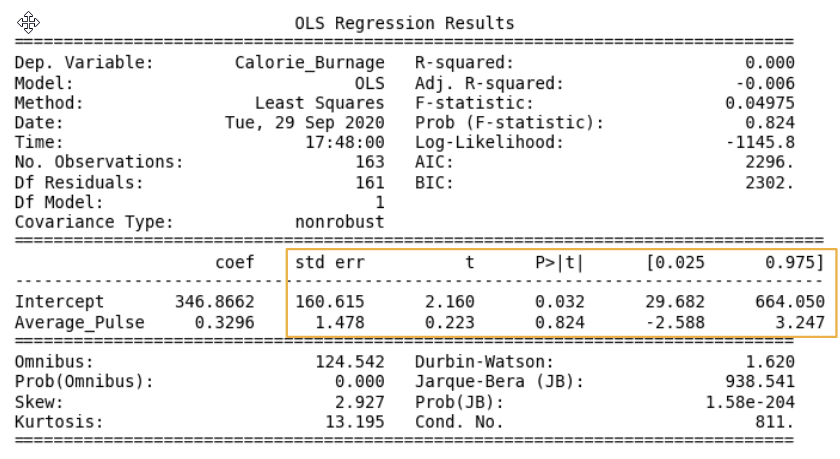# Data Science - Regression Table: P-Value

## The "Statistics of the Coefficients Part" in Regression TableNow, we want to test if the coefficients from the linear regression function has a significant impact on the dependent variable (Calorie_Burnage).

This means that we want to prove that it exists a relationship between Average_Pulse and Calorie_Burnage, using statistical tests.

There are four components that explains the statistics of the coefficients:

• std err stands for Standard Error
• t is the "t-value" of the coefficients
• P>|t| is called the "P-value"
•  [0.025  0.975] represents the confidence interval of the coefficients

We will focus on understanding the "P-value" in this module.

## The P-value

The P-value is a statistical number to conclude if there is a relationship between Average_Pulse and Calorie_Burnage.

We test if the true value of the coefficient is equal to zero (no relationship). The statistical test for this is called Hypothesis testing.

• A low P-value (< 0.05) means that the coefficient is likely not to equal zero.
• A high P-value (> 0.05) means that we cannot conclude that the explanatory variable affects the dependent variable (here: if Average_Pulse affects Calorie_Burnage).
• A high P-value is also called an insignificant P-value.

## Hypothesis Testing

Hypothesis testing is a statistical procedure to test if your results are valid.

In our example, we are testing if the true coefficient of Average_Pulse and the intercept is equal to zero.

Hypothesis test has two statements. The null hypothesis and the alternative hypothesis.

• The null hypothesis can be shortly written as H0
• The alternative hypothesis can be shortly written as HA

Mathematically written:

H0: Average_Pulse = 0
HA: Average_Pulse ≠ 0
H0: Intercept = 0
HA: Intercept ≠ 0

The sign ≠ means "not equal to"

## Hypothesis Testing and P-value

The null hypothesis can either be rejected or not.

If we reject the null hypothesis, we conclude that it exist a relationship between Average_Pulse and Calorie_Burnage. The P-value is used for this conclusion.

A common threshold of the P-value is 0.05.

Note: A P-value of 0.05 means that 5% of the times, we will falsely reject the null hypothesis. It means that we accept that 5% of the times, we might falsely have concluded a relationship.

If the P-value is lower than 0.05, we can reject the null hypothesis and conclude that it exist a relationship between the variables.

However, the P-value of Average_Pulse is 0.824. So, we cannot conclude a relationship between Average_Pulse and Calorie_Burnage.

It means that there is a 82.4% chance that the true coefficient of Average_Pulse is zero.

The intercept is used to adjust the regression function's ability to predict more precisely. It is therefore uncommon to interpret the P-value of the intercept.

W3Schools is optimized for learning and training. Examples might be simplified to improve reading and learning. Tutorials, references, and examples are constantly reviewed to avoid errors, but we cannot warrant full correctness of all content. While using W3Schools, you agree to have read and accepted our terms of use, cookie and privacy policy.